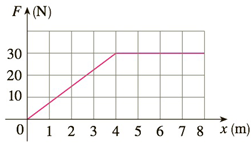Chapter 5.4, Problem 5E

Chapter
Section
Textbook Problem

# Shown is the graph of a force function (in newtons) that increases to its maximum value and then remains constant. How much work is done by the force in moving an object a distance of 8 m?To determine

To calculate:

The work done in moving the object to a distance of 8 m

Explanation

1) Concept:

Use the formula to calculate the work done.

2) Formula:

The work done in moving the object from a to b is given by

W=abf(x)dx

3) Given:

i. a=0 m and b=8 m

4) Calculation:

The force function F(x) is given in Newtons and the work is given in Joules

The area under the curve is given by

W=08F(x)dx

F  varies from 0 to 4 and remains constant on 4 to 8

From 0 to 4,Fx=304x and from 4 to 8,Fx=30

Therefore,

=04F(x)dx+48F(

### Still sussing out bartleby?

Check out a sample textbook solution.

See a sample solution

#### The Solution to Your Study Problems

Bartleby provides explanations to thousands of textbook problems written by our experts, many with advanced degrees!

Get Started

#### In Exercises 49-62, find the indicated limit, if it exists. 52. limx02x23xx

Applied Calculus for the Managerial, Life, and Social Sciences: A Brief Approach

#### Evaluate the integral. 63. xexdx

Single Variable Calculus: Early Transcendentals

#### In Exercises 27-30, evaluate each quantity. P(9,7)

Finite Mathematics for the Managerial, Life, and Social Sciences